# NCERT Solutions for Class 7 Maths Chapter 14 Symmetry Ex 14.2

NCERT Solutions for Class 7 Maths Chapter 14 Symmetry Exercise 14.2
Ex 14.2 Class 7 Maths Question 1.
Which of the following figures have rotational symmetry of order more than 1?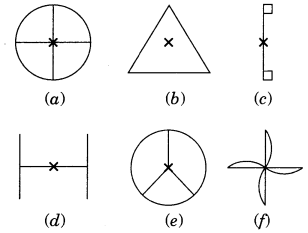Solution:
The figure (a), (b), (d), (e) and (f) have rotational symmetry more than 1.

Question 2.
Give the order of rotational symmetry for each figure:Solution:Let us take a point S on one end of the given figure. Rotating by 180°, S comes at other end and then again rotating by 180°, it comes at its original position.
∴ Order of rotational symmetry = $$\frac{360^{\circ}}{180^{\circ}}=2$$Let us take any point S in figure (1). It takes two rotations to come back to its original position.
∴ Order of rotational symmetry = $$\frac{360^{\circ}}{180^{\circ}}=2$$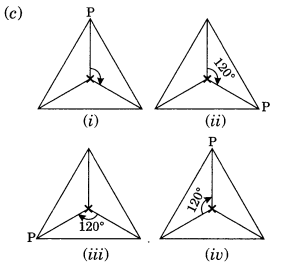Let us mark any vertex of the given figure. It takes three rotations to come back to its original shape.
∴ Order of rotational symmetry = $$\frac{360^{\circ}}{120^{\circ}}=3$$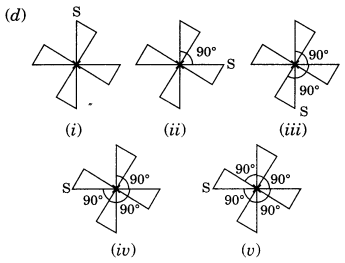Order of rotational symmetry = $$\frac{360^{\circ}}{90^{\circ}}=4$$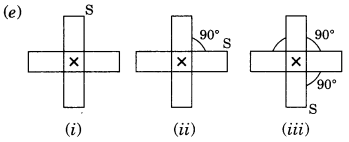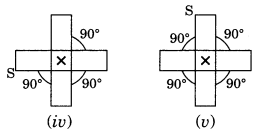∴ Order of rotational symmetry = $$\frac{360^{\circ}}{90^{\circ}}=4$$

(f) The given figure is a regular pentagon which can take one rotation at an angle of 72°.
∴ Order of rotational symmetry = $$\frac{360^{\circ}}{72^{\circ}}=5$$

(g) The given figure requires six rotations each through an angle of 60°
∴ Order of rotational symmetry = $$\frac{360^{\circ}}{60^{\circ}}=6$$

(h) The given figure requires three rotations, each through an angle of 120°.
∴ Order of rotational symmetry = $$\frac{360^{\circ}}{120^{\circ}}=3$$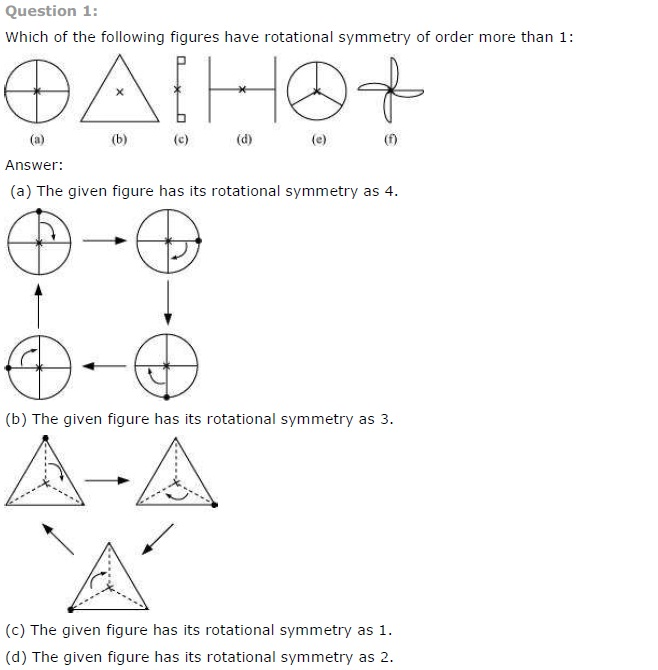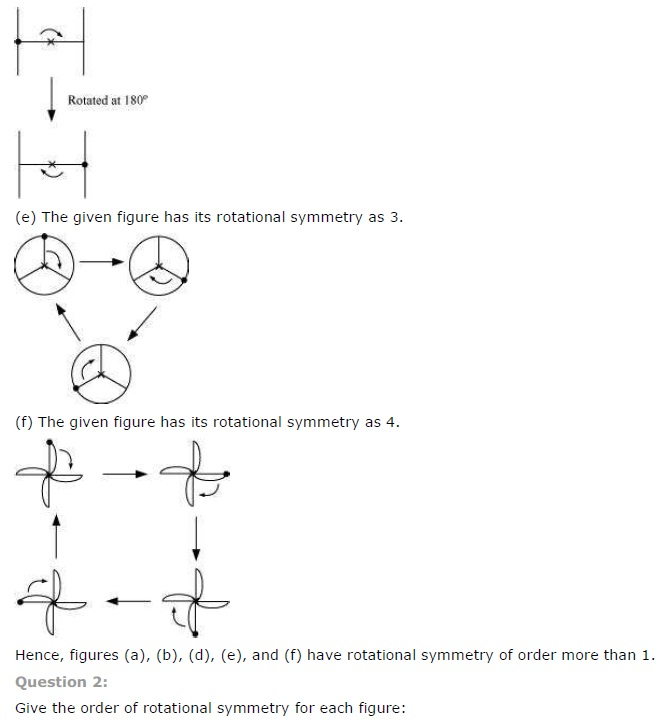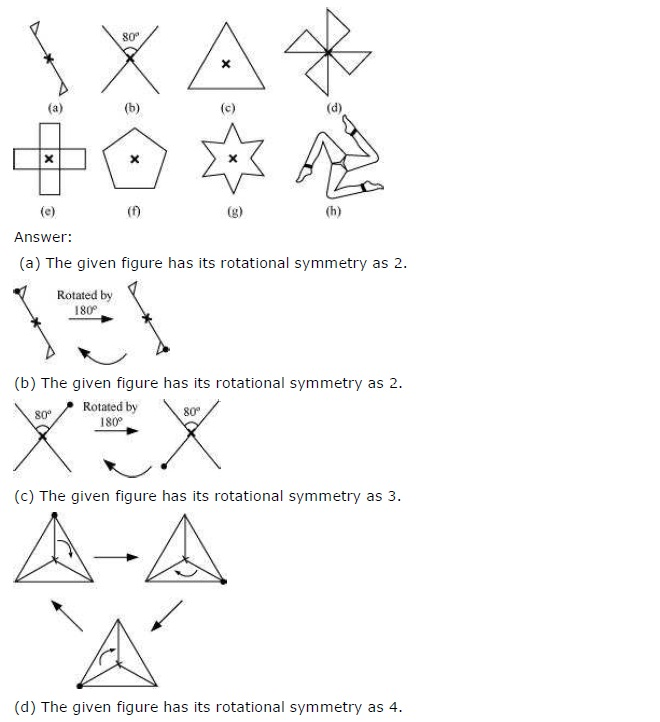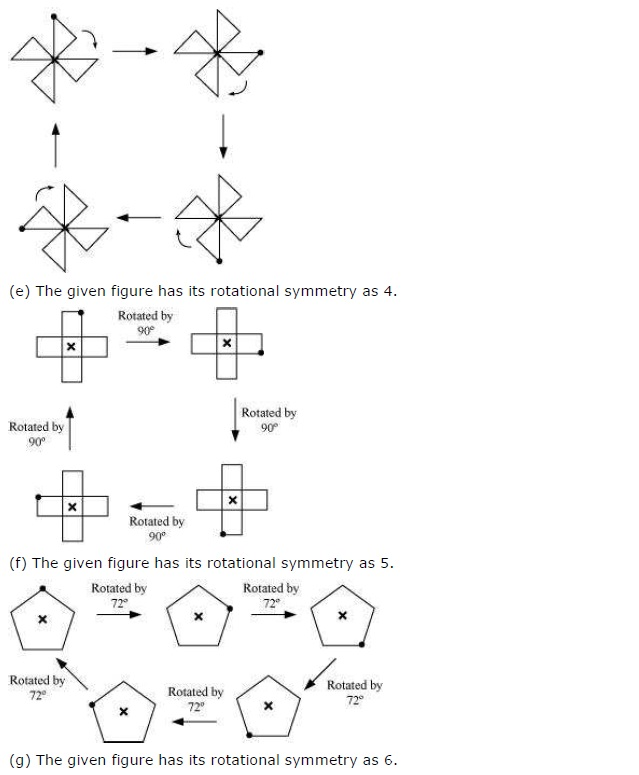+